# RD Sharma Solutions for Class 9 Maths Chapter 5 Factorization of Algebraic Expressions Exercise 5.2

RD Sharma Class 9 Mathematics Chapter 5 Exercise 5.2 Factorization of Algebraic Expressions is provided here. These exercise solutions are helpful to practice on the factorisation of algebraic expressions expressible as the sum or difference of two cubes. It will also help students score well in the examination. RD Sharma Solutions Class 9 chapter 5 are prepared by subject experts using simple and understandable language. Students can download RD Sharma exercise 5.2 by clicking on the link below.

## Download PDF of RD Sharma Solutions for Class 9 Maths Chapter 5 Factorization of Algebraic Expressions Exercise 5.2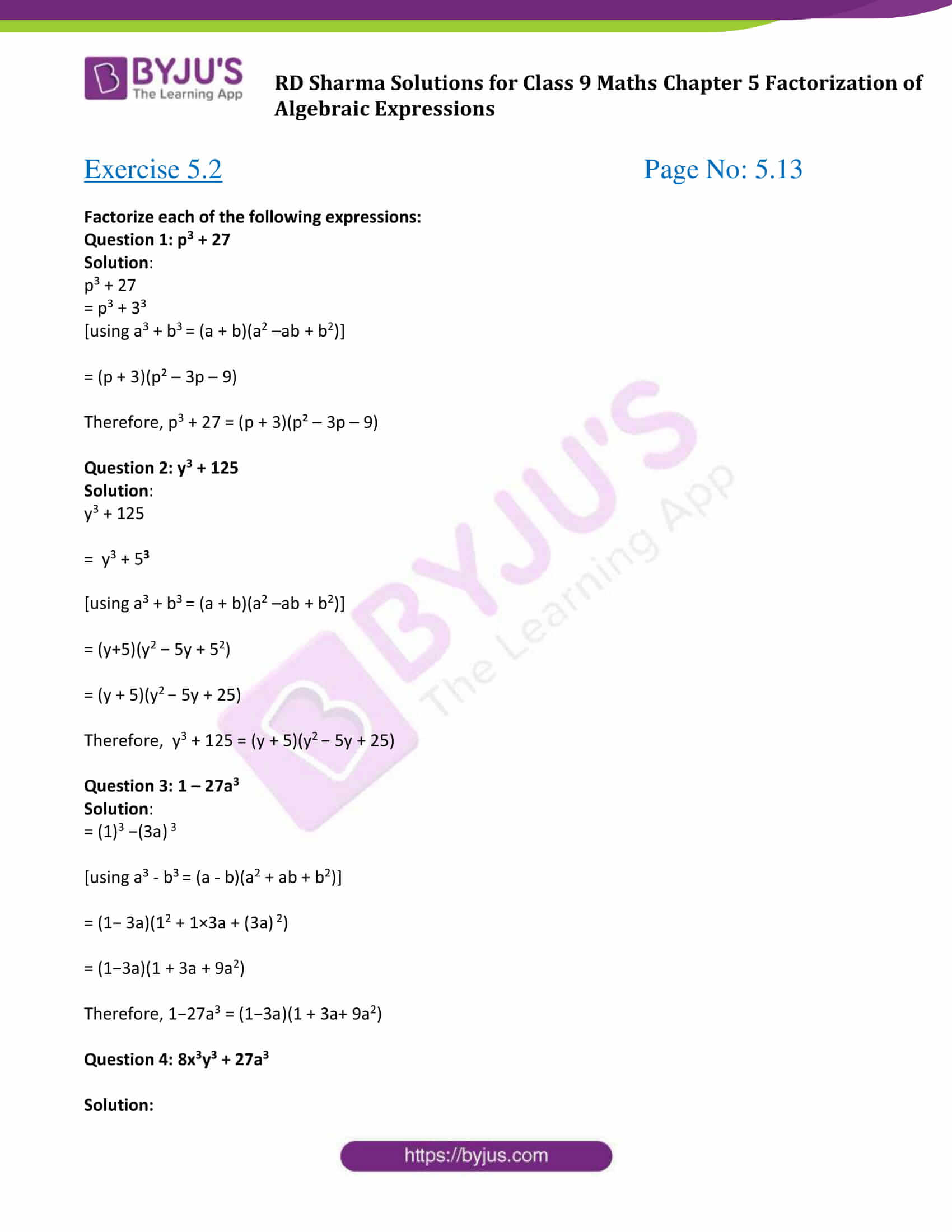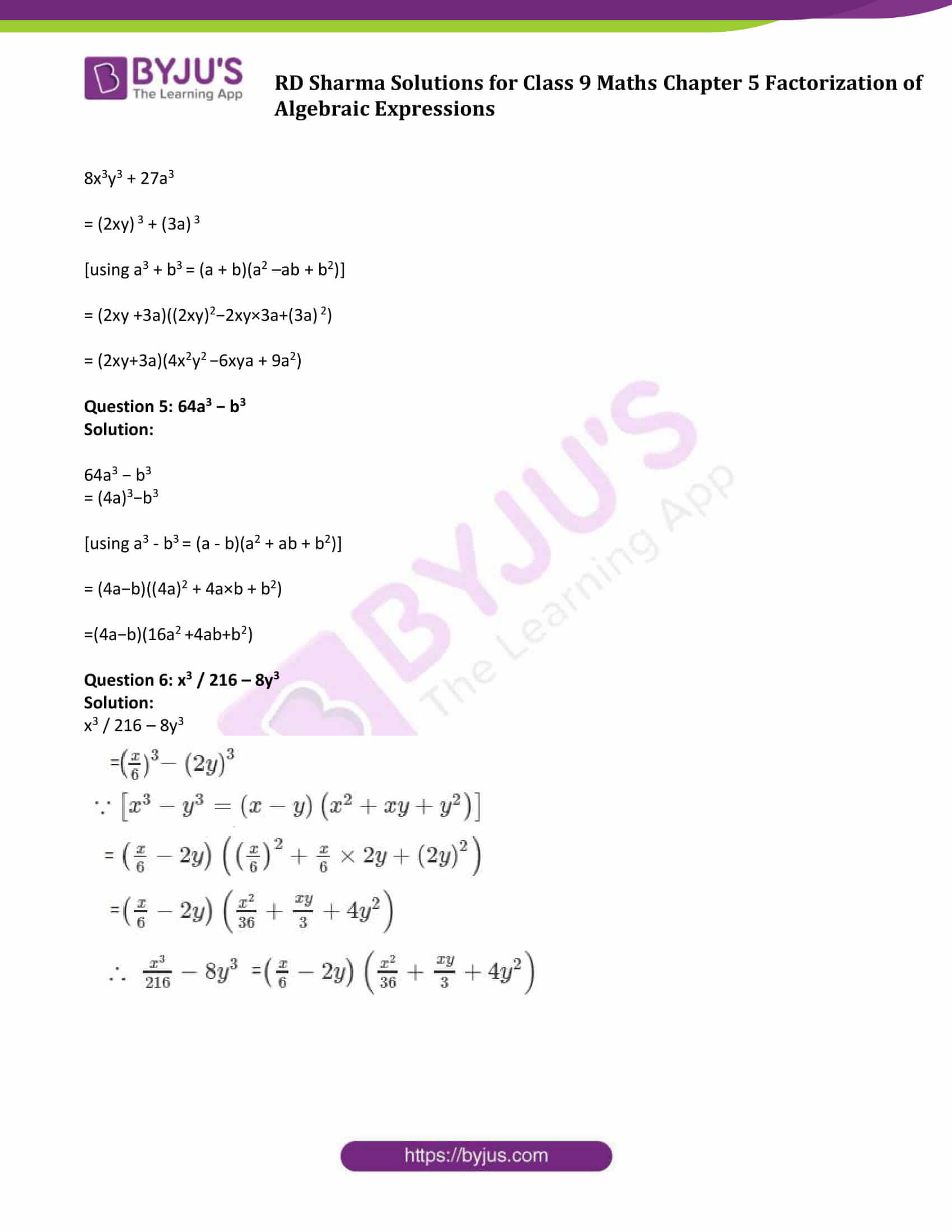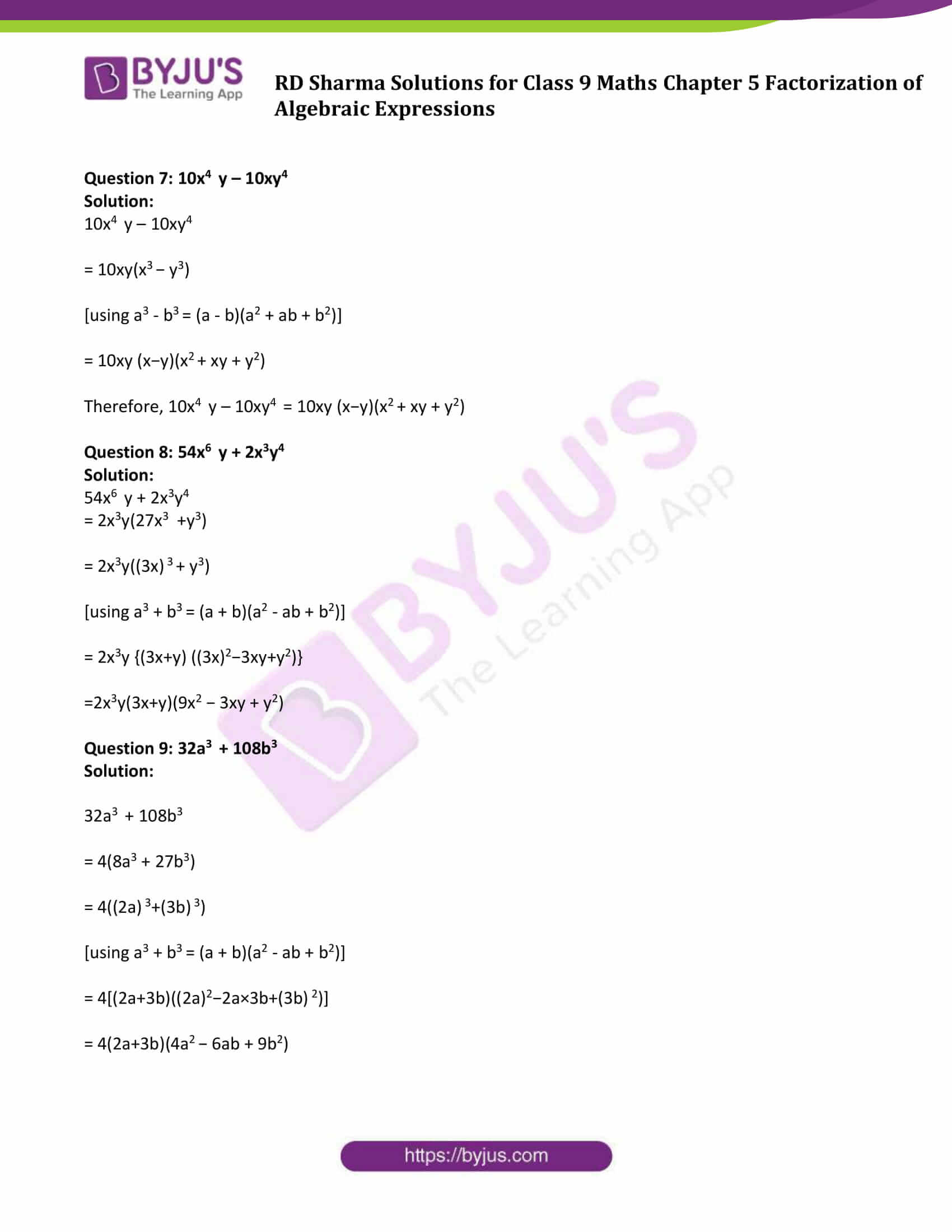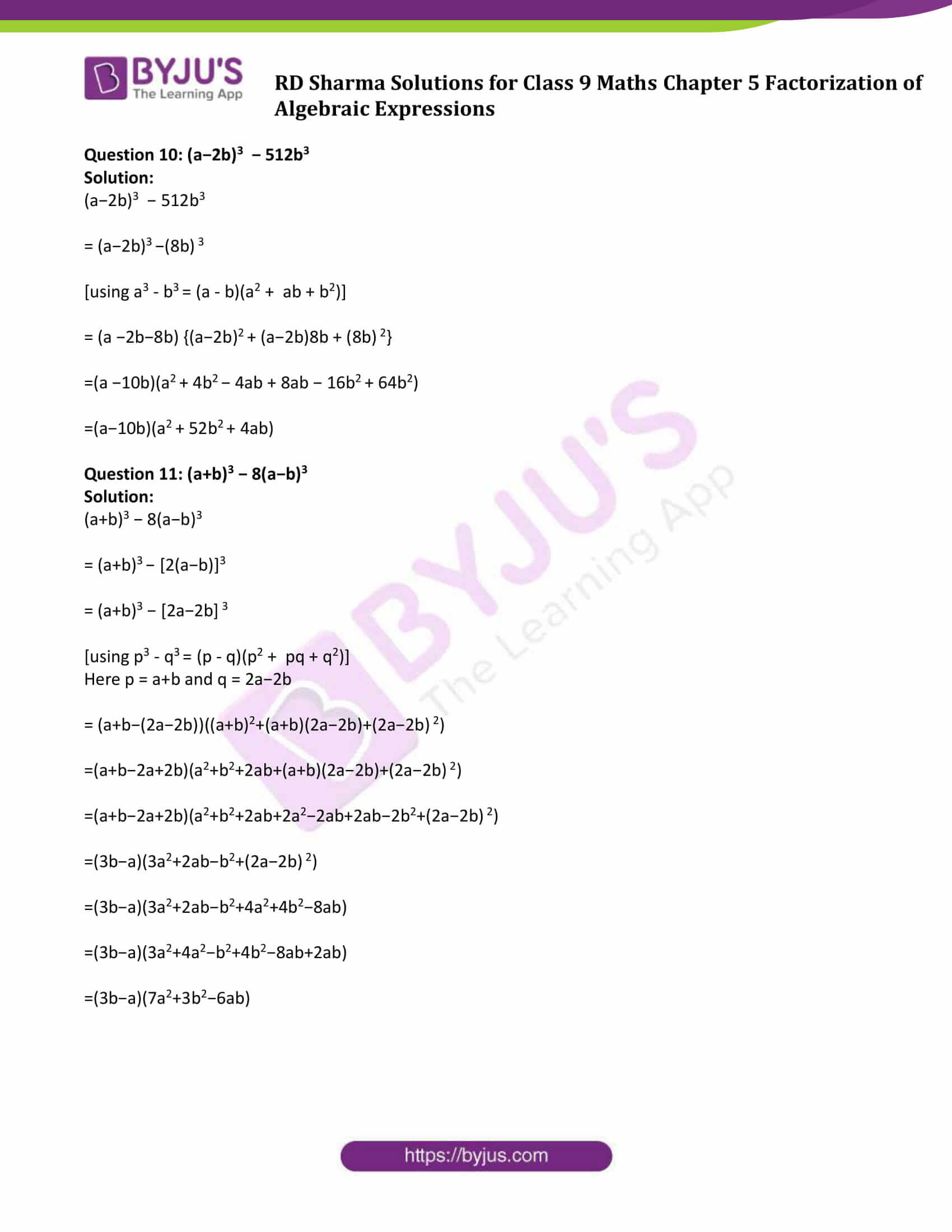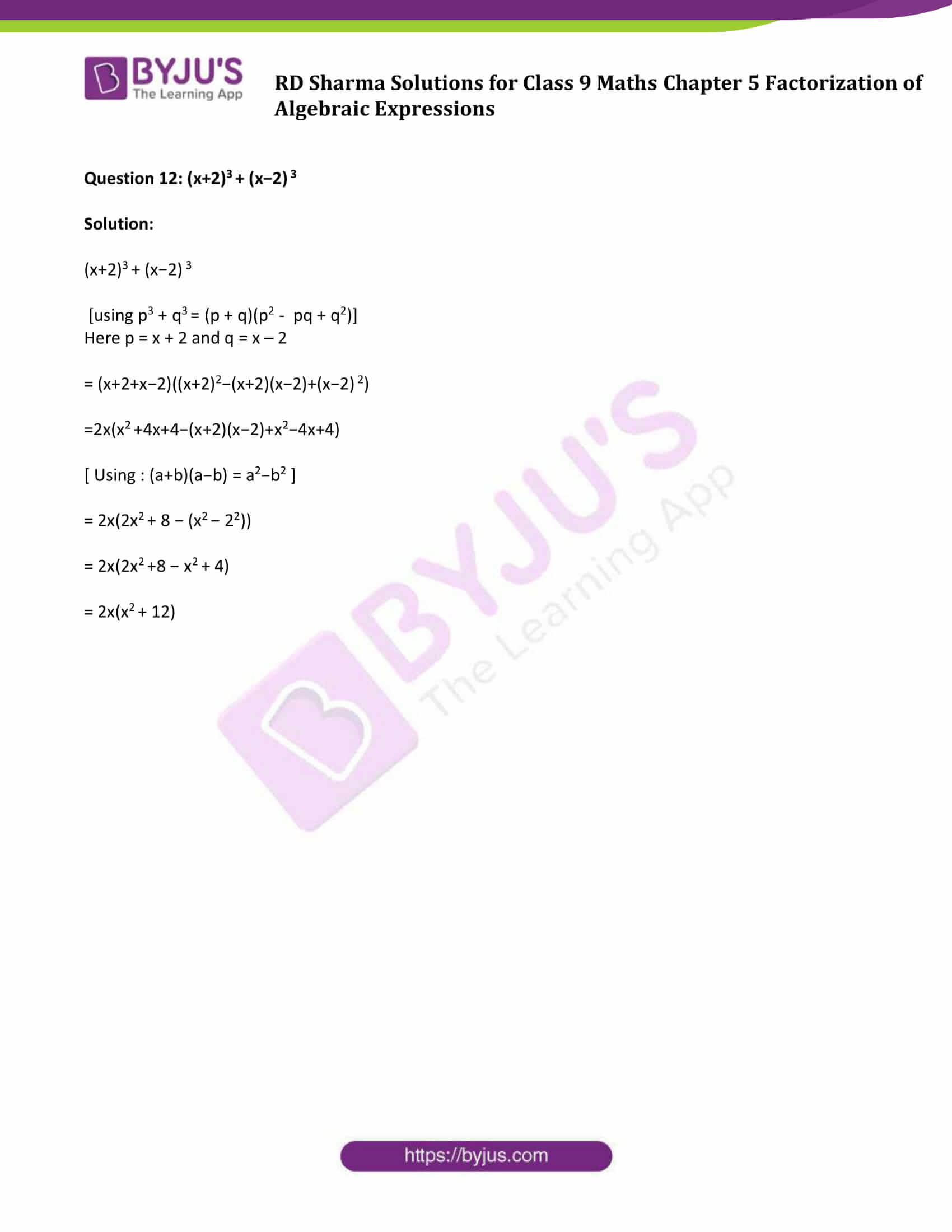### Access Answers to Maths RD Sharma Solutions for Class 9 Chapter 5 Factorization of Algebraic Expressions Exercise 5.2 Page number 5.13

#### Exercise 5.2 Page No: 5.13

Factorize each of the following expressions:

Question 1: p3 + 27

Solution:

p3 + 27

= p3 + 33

[using a3 + b3 = (a + b)(a2 –ab + b2)]

= (p + 3)(p² – 3p – 9)

Therefore, p3 + 27 = (p + 3)(p² – 3p – 9)

Question 2: y3 + 125

Solution:

y3 + 125

= y3 + 53

[using a3 + b3 = (a + b)(a2 –ab + b2)]

= (y+5)(y2 − 5y + 52)

= (y + 5)(y2 − 5y + 25)

Therefore, y3 + 125 = (y + 5)(y2 − 5y + 25)

Question 3: 1 – 27a3

Solution:

= (1)3 −(3a) 3

[using a3 – b3 = (a – b)(a2 + ab + b2)]

= (1− 3a)(12 + 1×3a + (3a) 2)

= (1−3a)(1 + 3a + 9a2)

Therefore, 1−27a3 = (1−3a)(1 + 3a+ 9a2)

Question 4: 8x3y3 + 27a3

Solution:

8x3y3 + 27a3

= (2xy) 3 + (3a) 3

[using a3 + b3 = (a + b)(a2 –ab + b2)]

= (2xy +3a)((2xy)2−2xy×3a+(3a) 2)

= (2xy+3a)(4x2y2 −6xya + 9a2)

Question 5: 64a3 − b3

Solution:

64a3 − b3

= (4a)3−b3

[using a3 – b3 = (a – b)(a2 + ab + b2)]

= (4a−b)((4a)2 + 4a×b + b2)

=(4a−b)(16a2 +4ab+b2)

Question 6: x3 / 216 – 8y3

Solution:

x3 / 216 – 8y3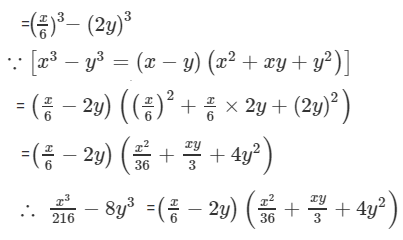Question 7: 10x4 y – 10xy4

Solution:

10x4 y – 10xy4

= 10xy(x3 − y3)

[using a3 – b3 = (a – b)(a2 + ab + b2)]

= 10xy (x−y)(x2 + xy + y2)

Therefore, 10x4 y – 10xy4 = 10xy (x−y)(x2 + xy + y2)

Question 8: 54x6 y + 2x3y4

Solution:

54x6 y + 2x3y4

= 2x3y(27x3 +y3)

= 2x3y((3x) 3 + y3)

[using a3 + b3 = (a + b)(a2 – ab + b2)]

= 2x3y {(3x+y) ((3x)2−3xy+y2)}

=2x3y(3x+y)(9x2 − 3xy + y2)

Question 9: 32a3 + 108b3

Solution:

32a3 + 108b3

= 4(8a3 + 27b3)

= 4((2a) 3+(3b) 3)

[using a3 + b3 = (a + b)(a2 – ab + b2)]

= 4[(2a+3b)((2a)2−2a×3b+(3b) 2)]

= 4(2a+3b)(4a2 − 6ab + 9b2)

Question 10: (a−2b)3 − 512b3

Solution:

(a−2b)3 − 512b3

= (a−2b)3 −(8b) 3

[using a3 – b3 = (a – b)(a2 + ab + b2)]

= (a −2b−8b) {(a−2b)2 + (a−2b)8b + (8b) 2}

=(a −10b)(a2 + 4b2 − 4ab + 8ab − 16b2 + 64b2)

=(a−10b)(a2 + 52b2 + 4ab)

Question 11: (a+b)3 − 8(a−b)3

Solution:

(a+b)3 − 8(a−b)3

= (a+b)3 − [2(a−b)]3

= (a+b)3 − [2a−2b] 3

[using p3 – q3 = (p – q)(p2 + pq + q2)]

Here p = a+b and q = 2a−2b

= (a+b−(2a−2b))((a+b)2+(a+b)(2a−2b)+(2a−2b) 2)

=(a+b−2a+2b)(a2+b2+2ab+(a+b)(2a−2b)+(2a−2b) 2)

=(a+b−2a+2b)(a2+b2+2ab+2a2−2ab+2ab−2b2+(2a−2b) 2)

=(3b−a)(3a2+2ab−b2+(2a−2b) 2)

=(3b−a)(3a2+2ab−b2+4a2+4b2−8ab)

=(3b−a)(3a2+4a2−b2+4b2−8ab+2ab)

=(3b−a)(7a2+3b2−6ab)

Question 12: (x+2)3 + (x−2) 3

Solution:

(x+2)3 + (x−2) 3

[using p3 + q3 = (p + q)(p2 – pq + q2)]

Here p = x + 2 and q = x – 2

= (x+2+x−2)((x+2)2−(x+2)(x−2)+(x−2) 2)

= 2x(x2 +4x+4−(x+2)(x−2)+x2−4x+4)

[ Using : (a+b)(a−b) = a2−b2 ]

= 2x(2x2 + 8 − (x2 − 22))

= 2x(2x2 +8 − x2 + 4)

= 2x(x2 + 12)

## RD Sharma Solutions for Class 9 Maths Chapter 5 Factorization of Algebraic Expressions Exercise 5.2

RD Sharma Solutions Class 9 Maths Chapter 5 Factorization of Algebraic Expressions Exercise 5.2 is based on the following topic:

• Factorisation of algebraic expressions expressible as the sum or difference of two cubes

a3 + b3 = (a + b)(a2 + b2 – ab)

a3 – b3 = (a – b)(a2 + b2 + ab)